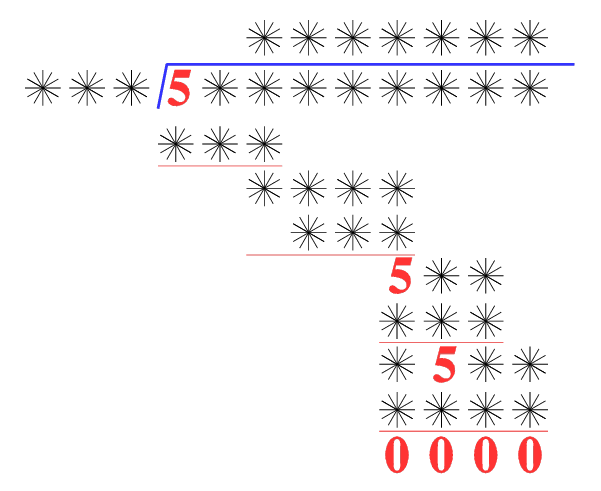#### You may also like### Even Up

Consider all of the five digit numbers which we can form using only the digits 2, 4, 6 and 8. If these numbers are arranged in ascending order, what is the 512th number?### Double Digit### What an Odd Fact(or)

Can you show that 1^99 + 2^99 + 3^99 + 4^99 + 5^99 is divisible by 5?

# Skeleton

##### Age 11 to 14 Challenge Level:

Amazing as it may seem the three fives remaining in the following `skeleton' are sufficient to reconstruct the entire long division sum.